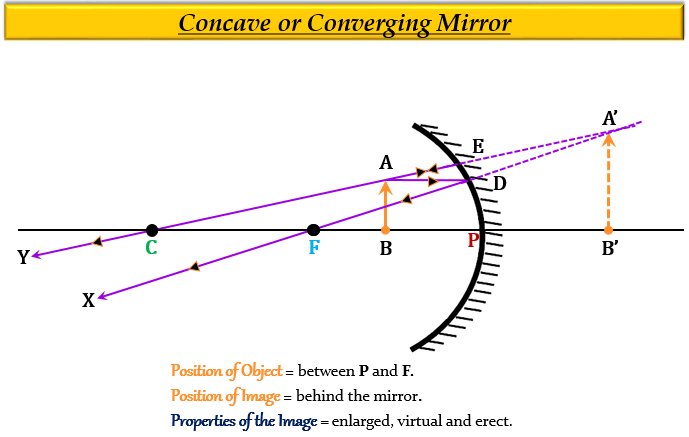# One of the following does not apply to a concave mirror. This is:(a) focal length is negative(b) image distance can be positive or negative(c) image distance is always positive(d) height of image can be positive or negative

(c) image distance is always positive

Explanation

The image distance $(v)$ is positive for a concave mirror only in the case when the object is placed between $(F)$ and $(P)$, because according to the "sign convention for spherical mirrors", all the distances measured from the pole $(P)$ of the mirror to the right side will be considered positive (as they will be in the same direction as the incident light). While all the distances measured from the pole $(P)$ of the mirror to the left side will be considered negative (as they are measured against the direction of incident light).

In this case, the image formed will be virtual and erect.Updated on: 10-Oct-2022

68 Views